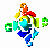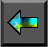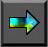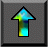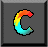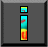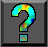Next: 4.5 Case Study: Tuple Space Up: 4 Putting Components Together Previous: 4.3 Performance Analysis

# 4.4 Case Study: Convolution

In the remainder of this chapter, we apply the modular design   techniques discussed in preceding sections in three case studies. We   start with an example from image processing, which we   use to study design tradeoffs that can arise when constructing   parallel programs from several components. We consider the problem of   applying a series of convolution operations to a sequence of images. Images, represented as arrays of size N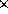N , are input in pairs on streams A and B ; convolution generates a new array of the same size that is output on stream C (Figure 4.4). A single convolution operation involves the transformation of two input arrays using independent two-dimensional fast Fourier transforms (2-D FFTs), a pointwise multiplication of the two transformed arrays, and the transformation of the resulting array using an inverse 2-D FFT, thereby generating an output array. A 2-D FFT performs 1-D FFTs first on each row and then on each column of an array. A 1-D Fourier transform,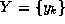, of a sequence of N values,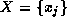, is given by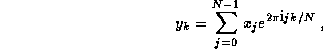where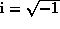. The FFT exploits symmetry to perform this computation insteps, each involving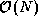operations.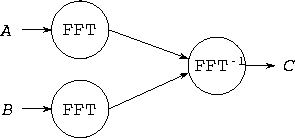Figure 4.4: Dataflow diagram for an image-processing pipeline. Two streams of images, A and B , are passed through FFT modules and then into an inverse FFT module, which first multiplies them and then applies an inverse FFT.

## 4.4.1 Components

We first consider the three components from which the convolution algorithm is constructed: forward 2-D FFT, multiplication, and inverse 2-D FFT. The pointwise multiplication is the simplest: Its communication requirements are zero as long as the arrays on which it operates have the same data distribution.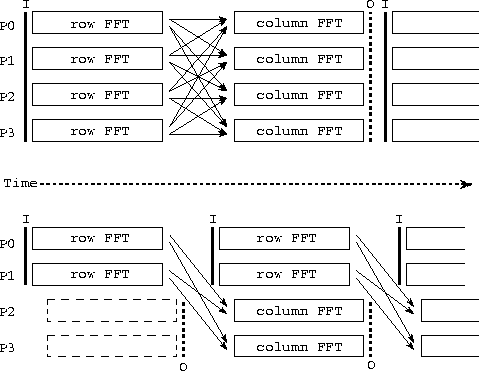Figure 4.5: Two parallel algorithms for computing a series of 2-D FFTs. In each case, the activity on each of four processors (P0--3) is shown over time, with arrows denoting communication and I and O denoting input and output operations. The algorithm illustrated in the upper part of the figure is a sequential composition of program components that perform 1-D FFTs, first on rows and then on columns of each input 2-D array; all-to-all communication is required to transpose the array after performing row FFTs and before performing column FFTs. In the second algorithm, data flow from a first set of processors performing row FFTs (P0, P1) to a second set performing column FFTs (P2, P3). Communication is required to move data from P0 and P1 to P2 and P3.

A variety of parallel algorithms are possible for the forward and inverse 2-D FFTs. A fine-grained algorithm can exploit concurrency within the 1-D FFTs performed on individual rows and columns of the input array, at the cost of considerable communication. A more coarse-grained algorithm performs independent 1-D FFTs in parallel, thereby avoiding a need for communication within the 1-D FFTs, but requiring communication when moving from a row-based to a column-based decomposition. We consider two algorithms based on the latter strategy. The first processes the input image stream sequentially, performing row FFTs and then column FFTs on each image in turn. The second algorithm pipelines the image stream, performing column FFTs for one image in parallel with the row FFTs for the next (Figure 4.5). These two algorithms are in effect sequential and parallel compositions, respectively, of code fragments that perform 1-D FFTs on the rows and columns of a two-dimensional array.

The first parallel algorithm is termed the transpose   algorithm, since it performs a series of one-dimensional transforms on   P processors while the array is partitioned in one dimension, then transposes the array and performs transforms in the second dimension using the same processors. The transpose operation requires that each processor send one message of size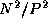to each of the P-1 other processors. Hence, total communication costs summed over P processors are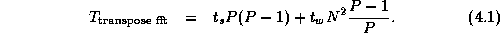The second algorithm is termed the pipeline algorithm, since it partitions processors into two sets of size P/2 which perform FFTs on rows and columns, respectively. Each processor in the first set must communicate with each processor in the other set, for a total of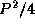messages. The entire array is communicated. Hence, total communication costs are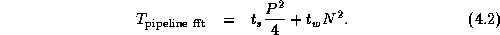Notice that communication costs are not necessarily distributed equally among processors in the second algorithm, since the sending and receiving processors form distinct groups. Nevertheless, Equations 4.1 and 4.2 give a rough idea of the relative performance of the two algorithms. The second algorithm sends significantly fewer messages and hence should be more efficient in situations in which message startup costs are dominant, for example, when N and/or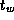are small or when P or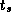are large. On the other hand, the first algorithm probably incurs lower data transfer costs and hence may be superior in other situations.

## 4.4.2 Composing Components

Having designed two alternative algorithms for the 2-D FFT, we now   consider the parallel convolution algorithm proper. Its four   components---two parallel 2-D FFTs, one matrix multiplication, and one inverse 2-D FFT (Figure 4.4)---can be combined using either sequential or parallel composition. If sequential composition is used, the parallel convolution algorithm can be represented as follows, with the fft and fft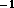calls invoking the transpose 2-D parallel FFT.

```
for each image

A= fft(A)

B= fft(B)

C= A.BC = fft(C)

endfor

```

If the input to this algorithm is decomposed appropriately (in one dimension, by rows), then because each FFT involves a transpose, total communication requirements are three times the cost of a single transpose: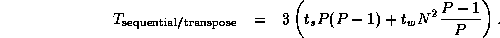Notice that because the forward FFT operates first on rows and then on columns, the inverse FFT must operate first on columns and then on rows, so as to avoid the need for an additional transpose operation between the forward and inverse FFTs.

If parallel composition is used, the three FFTs execute concurrently, each on one third of the available processors. (Because the   multiplication involves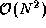rather than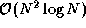operations, we regard it as insignificant and compose it sequentially with the inverse FFT.) Communication costing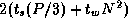is required to move data from the processors handling the forward FFTs to the processors handling the inverse FFT.

The 2-D FFTs within the parallel composition can be implemented by using either the transpose or pipeline algorithms, yielding two algorithm variants. Costs are as specified by Equations 4.1 and 4.2, except that each algorithm is executed three times, with P/3 rather than P processors involved in each execution. Combining these costs with the cost of the data movement between components, we obtain the following models.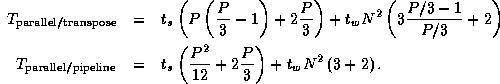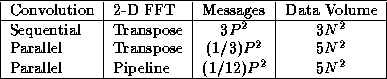Table 4.1: Approximate total message counts and data volumes for three parallel convolution algorithms, summed over P processors and assuming that P is reasonably large.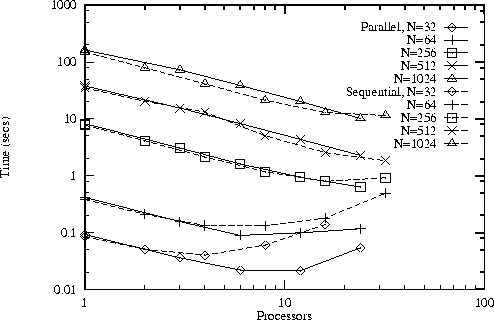Figure 4.6: Performance of the sequential/transpose (Sequential) and parallel/transpose (Parallel) convolution algorithms on an IBM SP computer for different problem sizes and numbers of processors. The latter algorithm is more efficient for smaller problems and larger numbers of processors.

The results of this brief analysis are summarized in Table 4.1. We see that the second and third algorithms perform fewer message startups but send more data. Hence, they can be expected to be more efficient on smaller problems and larger numbers of processors. This result can be confirmed experimentally, as illustrated in Figure 4.6. A flexible parallel program might incorporate all three algorithms and select the appropriate alternative at runtime. Of course, a programming tool that supports only sequential composition will allow only the first algorithm to be used.

## 4.4.3 Convolution Problem Summary

The convolution problem illustrates the design tradeoffs that can arise when constructing even relatively simple parallel programs. These tradeoffs can arise at multiple levels. First, we must identify candidate parallel algorithms for the component modules: in this case, the 2-D FFT. Then, we must decide how to compose these building blocks so as to construct a complete parallel algorithm. Aspects of the complete design in turn influence the techniques used within components, requiring for example that we operate on columns before rows in the inverse FFT. The performance analysis must take into account all these design decisions.Next: 4.5 Case Study: Tuple Space Up: 4 Putting Components Together Previous: 4.3 Performance Analysis